# Container Numbers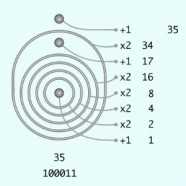This page is a tutorial on how iconic container numbers work. Addition is putting outside, while multiplication is putting inside. The container numbers on this page are expressed as unit-ensembles with depth-value. The Unit-ensembles page describes how to represent numbers as collections of identical tokens. The Depth-value Notation page shows how enclosures can be used to group units.  Container numbers represent magnitude by enclosing units in containers, that is, by drawing circles, boxes, or other enclosures around units.  Each container multiplies the value of its contents by the base of the number system.  The base is usually 10 (the decimal system) or 2 (the binary system).  Here we will show binary container numbers in order to focus on how iconic arithmetic works.. The Image shows the binary container number 35. Both decimal and binary container numbers are illustrated in the Iconic Calculator section.  The Container Algebra page has a more complete discussion of the algebraic structure of container numbers. Finally, Network numbers work just like container numbers, but network numbers use signal propagation rather than containment as an active model of computation. Block numbers also work like container numbers, but using yet another type of spatial representation, three-dimensional stacks of blocks.

Three different container notations are used on this page.  Parens notation is a textual representation that uses parentheses as delimiting tokens to stand in place of surrounding containers.  Circle notation is a graphic two-dimensional form that represents containers by surrounding circles and ovals.  For example, the image shows how to read the binary container number 35 when it is represented in circle notation. The primary representation is dynamic circle notation, which shows the process of computing with container numbers. Dynamic circle notation shows how arithmetic works. The symbolic numbers that are used universally in our culture do not permit dynamic interaction, they cannot illustrate the mechanism of addition or of multiplication.  Instead, symbolic numbers rely on memorization of number facts and the abrupt substitution of results in place of operations.  We write 3+4=7, but we have no way to see or to experience what adding three to four means or how it happens.  Iconic numbers, however, show and explain the processes of addition and multiplication.  Iconic numbers can be manipulated physically, permitting us both to watch computation taking place and to participate actively in the operations of arithmetic. Symbolic numbers expressed in place-value notation require a sequential step-wise approach to arithmetic.  By escaping the tyranny of the one-dimensional textual line, iconic numbers let us do many operations, or “steps”, at the same time.  As a consequence, iconic algorithms are much simpler than symbolic algorithms.

In the following, doing arithmetic is illustrated by video animations of counting, adding, subtracting, multiplying and dividing using a two-dimensional version of container numbers.  The animations not only show how container arithmetic works, they show how arithmetic itself works. The intent of the tutorial is to provide models of activity, not passive viewing experiences. The animations show what to do in order to do arithmetic. The Parens Notation page includes the parens form of each of the dynamic container arithmetic examples shown in the videos. The parens form shows the skeleton outline of the animated transformations, bridging the gap between conventional symbolic representation and the dynamic interaction of the iconic representation. The Parens Notation page also describes the alignment between one-dimensional sequential and two-dimensional parallel computation.

Four 2006 videos that compile the animations on this page are available for download. All four are VGA (640×480) size in .MP4 format.

Container Number Operations (3 1/2 minutes, 3Mb) shows condensed animations of reading and the four basic operations (+, –, x, ÷).

Container Numbers (8 1/2 minutes, 6Mb) shows most of the animations on this page.

Spatial Arithmetic (11 minutes, 26Mb) is a narrated tour through the animations on this page.

## Containers

Containers are a natural iconic form, they look like what they do.   Iconic containers represent the single concept of containment, distinguishing what is inside and what is outside.   Although physical containers have properties such as shape and size, iconic containers are abstract in that we do not associate any properties with them other than containment.  Without an underlying size or shape, any iconic container can contain any other container.  But a container cannot contain itself, it is not possible to put a box inside itself for example.  A container can contain any collection of other containers.  It’s possible for a container to contain nothing, or to contain one other object, or to contain many other objects.

Although containers are two and three dimensional forms, they can also be represented typographically by delimiters, such as parentheses ( ), square brackets [ ], curly braces { }, and less-than and greater-than tokens < >.  For one-dimensional containers, we will use parentheses, called parens.

Container numbers represent a unit as a container that is full.  To make this distinction, a unit is represented by a solid circle , nothing more can be inside.  When used within binary numbers, parens double their contents, so that (•) represents two units.

The depth-value notation represented by nested containment does not have the same limitations as place-value notation. In particular, each container is independent of the others.  There is no reliance on the position of a digit in a form, nested containers take care of magnitude by structure rather than by an arbitrary placement convention.  When addition is represented as putting things into the same container, both the Additive Principle and the natural parallelism of unit-ensembles is maintained.

Any particular number has many different iconic representations depending upon how much it has been condensed from unit-ensemble notation to container notation.  For example, some of the ways we can write the number 6 are: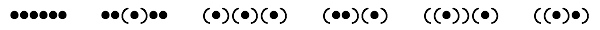There are two canonical ways to represent iconic numbers, as units only (hard to work with) or as maximally nested (easy to work with).  The effort in using container numbers is in simplifying them to a standard nested form.  The operations of addition and multiplication of container numbers then require very little effort, both are achieved by solely putting numerals in particular containers.

These techniques were first published in 1995 for a one-dimensional sequential version of base-2 container numbers by Louis Kauffman of the University of Illinois at Chicago.  Spencer Brown Numbers presage the iconic representation and manipulation of whole numbers.

This video shows the binary container numbers from 0 to 16.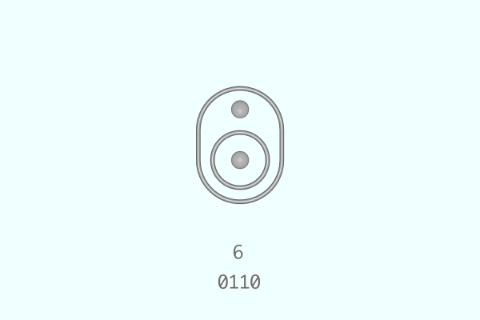Counting from 0 to 16 with binary container numbers

The content of this animation is laid out in a strip here, so that the entire sequence can be seen at the same time.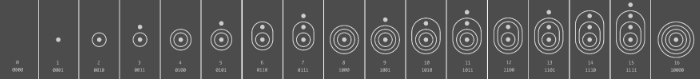To read a binary container number, begin with the deepest nested unit.  Crossing outward over a boundary doubles the accumulated value.  This video shows the doubling effect of container boundaries.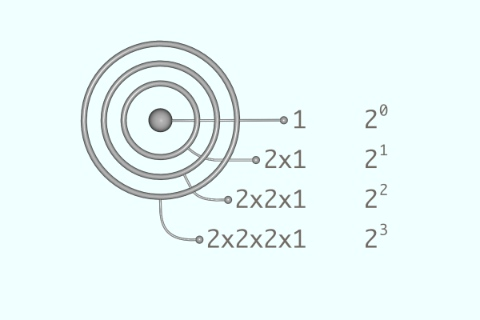Enclosures multiply their contents by two

When crossing into a new space, if there is a unit in that space, then add one.  This is the Additive Principle at work, objects in the same space add together.  Here are three videos that illustrate reading the container numbers 3, 12, and 35.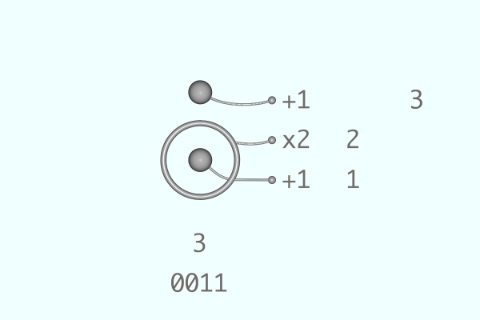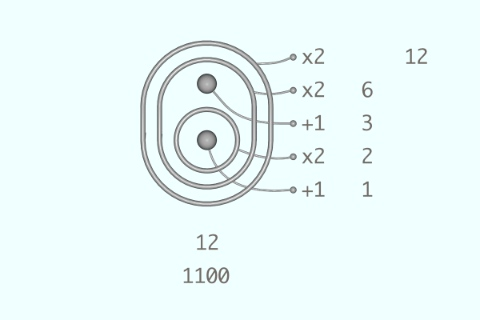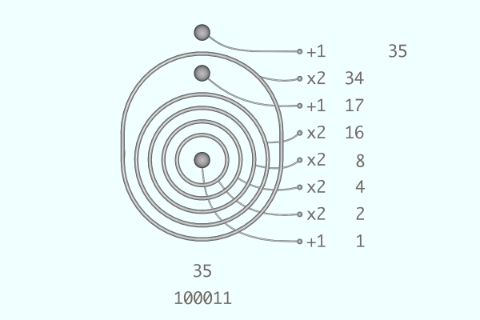## Standardizing Container Numbers

The purpose of standardization is to make numbers easier to work with. Of course there are many ways to define what it means to be easier to work with. We’ll initially adopt a criterion of minimal structural complexity, based on the original motivation to make it easier to read the cardinality, or exact size, of large ensembles.

Three simple transformation rules are used to convert container numbers into their standard nested minimal form, technically called a normal form.  The Group Rule collects units into groups.  For binary numbers, each group consists of two units.  For decimal numbers, each group consists of ten units.  The Merge Rule joins containers that share the same space.  The Cancel Rule deals with negative numbers.

Units are collected into groups by the Group Rule, which converts a pair of units into one unit inside an enclosure.  The enclosing container multiplies its contents by two.  In standard notation, this rule is 1+1=2*1.  The Group Rule in parens notation is followed by  an animation showing the dynamic application of the rule.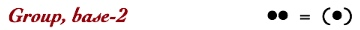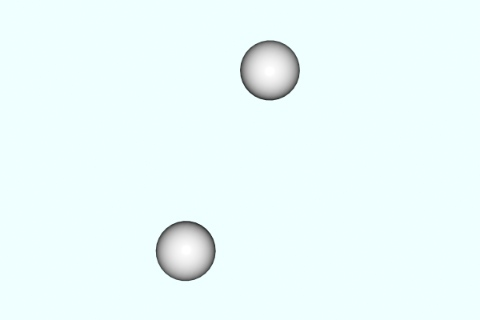The Group units action

Iconic grouping collects units into ensembles of a specific size (the base), and represents each group by a boundary around one unit. Boundaries in the same space merge by combining their contents.  In standard notation, the Merge Rule is the distributive property, na+nb=n(a+b), where n is the base of the number system. The Merge Rule in parens notation is followed by an animation showing the dynamic application of the rule.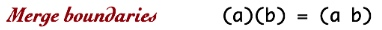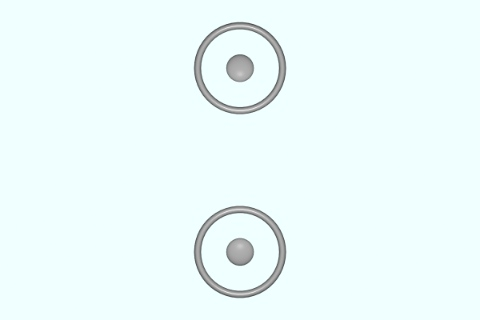The Merge boundaries action

Positive units are represented by a full circular container.  Negative units are represented by the arbitrary choice of a pentagon.  The Cancel Rule deletes positive and negative units in pairs.  In standard notation, this rule is 1+(–1)=0.  The Cancel Rule in parens notation is followed by an animation showing the dynamic application of this rule.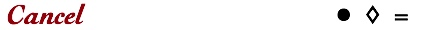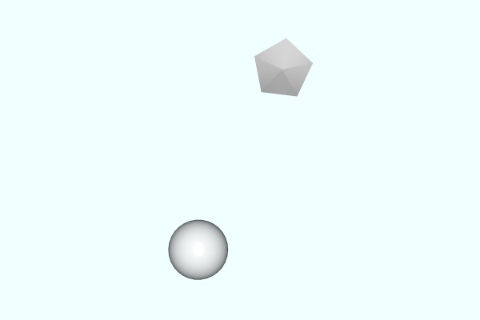The Cancel opposite units action

Putting-into-the-same-space, grouping units, merging containers, and canceling opposites are all manifestations of the Additive Principle. Putting-into-the-same-space is, in fact, the definition of the Principle. Standardization is motivated by our criterion to minimize structure, so that all three standardization transforms remove structure. Grouping and Merging both reduce two separate forms into one. Grouping defines a specific collection of units and puts them together in their own space as an ensemble, while at the same time transferring the cardinality or multiplicity of the collection onto a single unit within the newly bounded space. ••=(•) Merging puts containers together following a different rule. We are counting units not containers, so it doesn’t matter how many containers merge together at the same time. Units group rather than merge since they are already full. Containers don’t count so they cannot group together; containers merge. Canceling is yet another putting together. Since we have defined negative units as holes and positive units as wholes, when they are put together, nothing is left.

Technically, as an aside, space cannot support any type of relation, since we have given it no structure. It is always the container and not the contained space that defines or implements transformations. This very important principle assures us that only existant forms have properties. It can be made notationally explicit by writing a localization container around each transformation. We’ll use explicit brackets here but render them implicit and invisible throughout the other sections. That turns the phrase “put into the same space” into “put into the same container”. A localization container is an imaginary rather than an actual boundary — it doesn’t multiply, rather its purpose is to draw attention and to assure that all forms have an outermost container.

Here are the three standardization rules with the localization boundary made explicit. I’ll use a white lenticular bracket because it draws attention.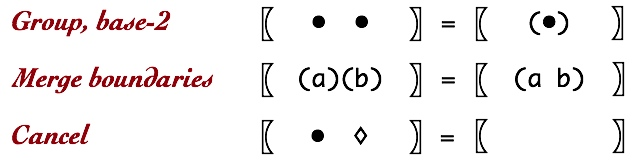To add, put numbers together. Group and Merge then take over to simplify the numbers into the nested form of the sum.  To illustrate Group and Merge operating in concert, the next animation shows four single units being converted into the nested form of the number 4.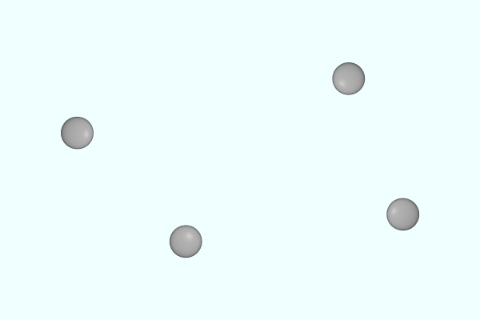Standardizing four units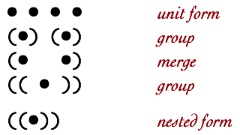It is sometimes convenient to record the progress of the dynamic simplification process using text.  Parens notation provides a typographical representation that shows each transformation step of an iconic number.  The parens sequence on the right is read vertically, each line replicates a step taken in the animation going from the unit-ensemble form to the fully nested form. Parens notation provides a static outline of the transformation sequence.

Here is another dynamic addition, the parens form is in the Supplement.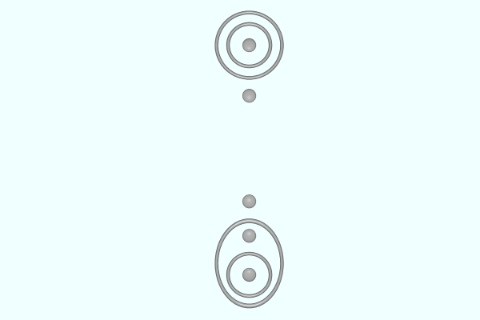Here is the content of this animation laid out as a strip, so that the entire sequence can be seen at one time.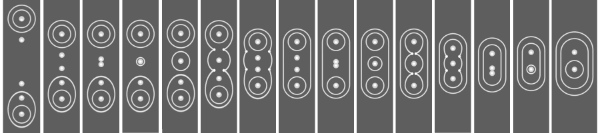## Subtraction

To subtract, add positive and negative numbers and then cancel pairs of opposites.  Often a nested unit must be brought to the same level of nesting as a canceling unit, the container analog to borrowing.  To do this, the Group Rule is run backward, as Ungroup.  This animation shows an enclosed unit ungrouping.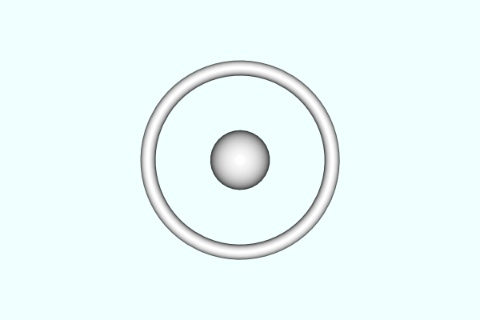The Ungroup action

To facilitate the cancellation of deeply nested units, it is sometimes also necessary for the Merge Rule to run backwards as Unmerge.  Here is an animation illustrating unmerging, the deconstruction of an enclosured unit, (•), into the two individual units it represents.The Unmerge action

To put Ungroup in context, here is the video of 2–1=1, which is more accurately expressed as 2+(–1)=1: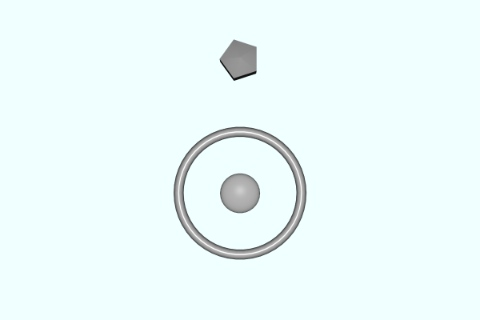The Group and Merge operations of iconic arithmetic are the same for both positive and negative numbers, they are independent of type of unit.  This video shows 1–2=–1, that is, 1+(–2)=–1.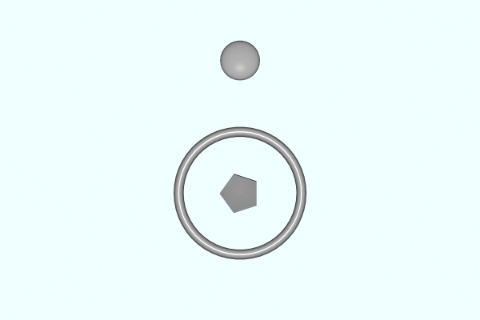As illustrated in the next animation of 2+(–2)=0, positive and negative numbers merge boundaries prior to inner units canceling. The cancel rule only applies to units sharing the same space.  When an enclosure is empty, it too will vanish.  Absence or emptiness in iconic arithmetic means zero.  It is both simpler and more intuitive to have no symbol for nothing than it is to use 0.  Since an enclosure multiplies its contents by two, an enclosure of nothing is multiplying 0 by 2, resulting in 0.  So the enclosure itself, since it is equal to 0, vanishes.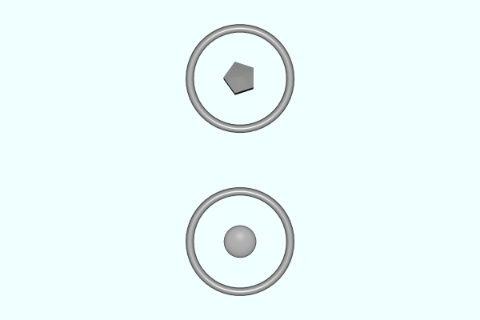The addition 7+5=12 was illustrated above. When two negative numbers are added together, the process is exactly the same, but the individual units are negative rather than positive.  This animation of (–7)+(–5)=–12 shows that there is no mystery in negative numbers, they add just like positive numbers.  The confusion of locating negative numbers on a one-dimensional number line, and then essentially trying to add backwards is avoided, as is the difficulty of trying to explain (often with banking metaphors) that negative seven minus five yields negative twelve, –7–5=–12.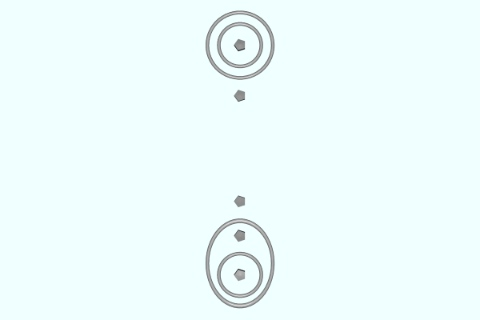The next two videos show 7–5=2, that is, 7+(–5)=2, and 5–7=–2, that is, 5+(–7)=–2.  The two animations are identical, except that the type of units for each number is exchanged.  These animations emphasize that adding and subtracting are the same process, independent of the type of unit. A consequence is that conventional addition and subtraction operations can be mixed together with container numbers.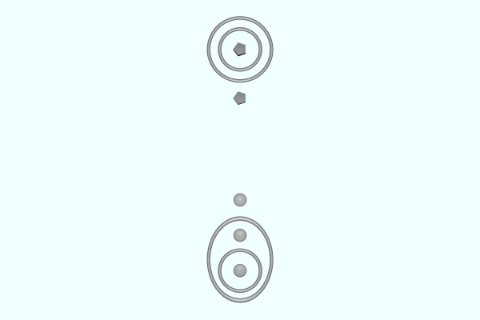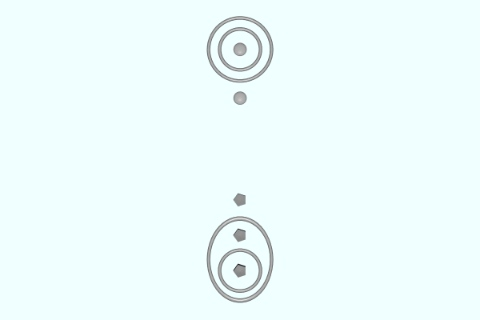## Multiplication

Multiplication is the replacement of the units of one number by another entire number.  This process is stated as substitute number-A for each unit in number-E.  Square brackets serve as an abbreviation for this action.  Substitute A for in E is written as [A • E].  The number substituted into is being multiplied by the number being substituted, so the above substitution is read as E multiplied by A, or  E*A.

Once substitution has occurred, the two numbers have been multiplied.  Then Merge and Group take over to simplify the form of the result.  In the simple case of replacing by itself (which is written as [• • E] and is read as Substitute for in E), nothing changes.  The animation shows 3×1=3, abbreviated as the substitution [• • 3].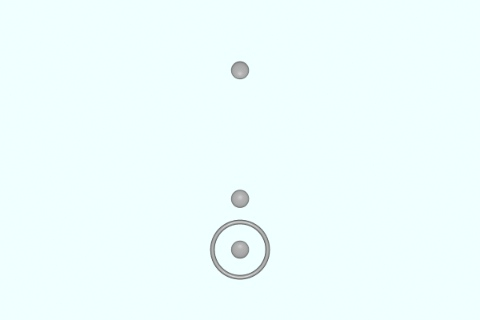Multiplying 3 by 1

In contrast, the next video shows 1×3=3, that is [3 • •].  The unit is replaced by the iconic number 3.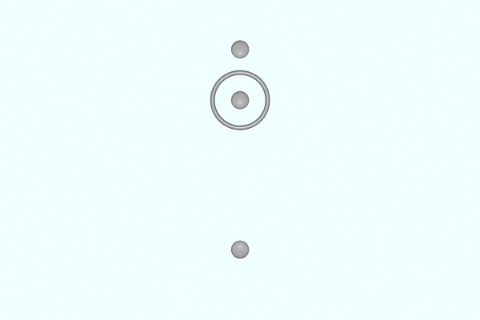Multiplying 1 by 3

The commutativity of multiplication, that 1×3=3×1, becomes a commutativity of substitution. The axiomatic approach to substitution is described on the Container Algebra page.

When 2 is multiplied by 3, the single unit within iconic 2 is replaced by the iconic number 3.  The result, 6, requires no further simplification, as this animation of 2×3=6 as the substitution [3 • 2] shows.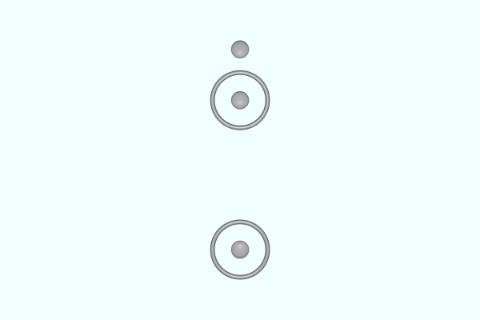Multiplying 2 by 3

When 3 is multiplied by 2, both units in iconic 3 are replaced by the iconic form of 2.  This result requires one application of Merge to simplify.  The animation of 3×2=6 as [2 • 3] is next.Multiplying 3 by 2

The next four animations show 5×7=35 as [7 • 5] and 7×5=35 as [5 • 7]. The first two videos show that directly after substitution, the intention to multiply has been achieved.  Although each result of the substitution is initially different, both are a representation of 35.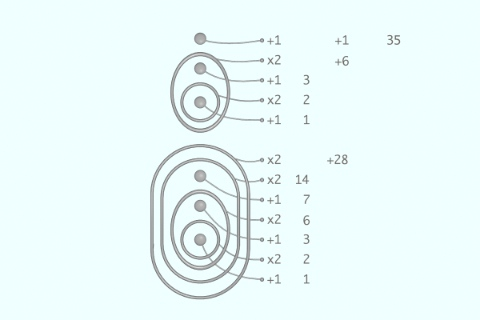Verifying that substituting 7 into 5 is 35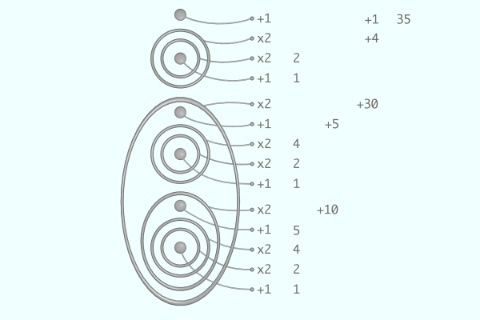Verifying that substituting 5 into 7 is 35

When Group and Merge are applied to each initial multiplication, the two forms quickly converge to a single common form that then continues to simplify until the final nested form of 35 is achieved.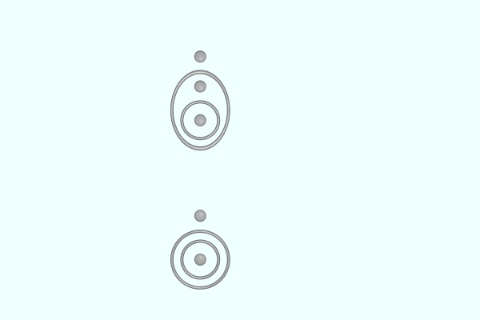Multiplying 5 by 7

Below, the content of this animation is laid out in a strip so the entire animation can be seen at one time.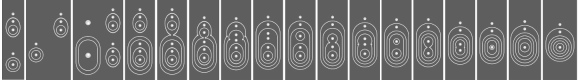For comparison here is the animation of 7×5. The convergence of these two sequences is clearly illustrated on the Parens Notation page, under the parens form for the pair of multiplications.Multiplying 7 by 5

Multiplication by negative numbers is discussed on the Container Algebra page.

## Division

Iconic division is the inverse of multiplication, Unsubstitution so to speak. However, the general operation of substitution itself is sufficient to incorporate the concept of division.  This animation shows 2÷2=1 as the substitution [• 2 2]. In the animation, miniature icons are used as placeholders.Division of 2 by 2 using substitution

This elementary case illustrates that division is also a type of substitution. The iconic form of two is first identified within the form to be divided and then replaced by a unit.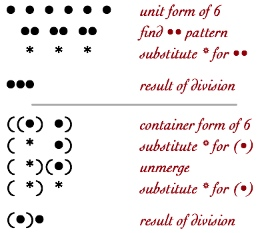This can be written as [• 2 2]: Substitute a unit for every 2 in 2.  In general, E÷A is [• A E]: Substitute a unit for every A in E.

To divide 6 by 2, we would substitute a unit for every two units in the number six. This is illustrated in parens notation for unit-ensembles. Groups of two units are located within the unit-ensemble for six, and each is replaced by a unit. It is convenient to use a place-holding asterisk, *, as a unit to avoid confusion with the original units.  The result is the unit-ensemble three. The same principle applies to container numbers.  Whenever an iconic 2 is located within 6, a unit is substituted. This is also illustrated in parens notation.  The iconic meaning of 6÷2 is the action: Substitute  for 2 in 6, which can be abbreviated as [• 2 6].   The next animation illustrates the dynamic division of the container number 6 by 2.Dividing 6 by 2

The final two animations show 35÷5=7, written as [• 5 35], and 35÷7=5, written as [• 7 35].  In both cases the container number 35 is decomposed until the form of 7 (respectively the form of 5) is identified.  There is no strategy required, decomposition via Ungroup and Unmerge of the innermost container combined with partial pattern-matching of the target form 7 (respectively 5) is sufficient.  The parens version of these videos in the supplement show the decomposition sequence.Dividing 35 by 5Dividing 35 by 7

The Parens Notation page shows an alternative textual notation for the dynamic containers in each of the videos.